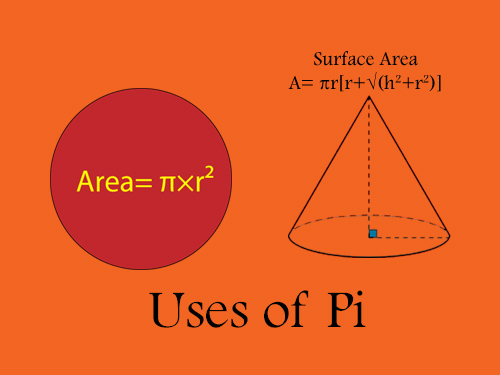# What is pi and why is it significant?

Published on 24-Aug-2022

## What is pi?

Pi is a huge part of the world of mathematics. It starts with 3.14159265358979323... and there are billions of more digits of pi! Yes, it's enormous when we begin to write its value, so we use the symbolic form, π. 'Infinity' is the perfect term to describe the senseless procession of pi. It has been calculated to be over 1 trillion digits beyond its decimal point. It turns out to be an irrational number as the exact value is unknowable.

There are a lot of essential as well as fun facts out there regarding pi. Pi is ancient, as we all know, and it's all-encompassing, which conveys the endless procession of digits. Many people surprisingly could memorize the vast stretches of it, which is not an easy task. In many books and movies, there's a small part of pi. There's a day dedicated to pi. Pi Day is on March 14, an annual celebration of the mathematical sign pi. The first three digits of π are 3,1 and 4, and that's why it is celebrated on March 14. In ancient times, it was used as a secret code for some confidential works by ancient mathematicians.
Isaac Newton, the English mathematician and physicist, used infinite series to compute pi to 15 digits in 1665, and German mathematician Gottfried Wilhelm Leibniz discovered it. Archimedes of Syracuse (287-212 BC), one of the greatest mathematicians, did the first calculation of π.

## why is it significant?

Pi is the ratio of the circumference of a circle to its diameter, which became a new unit of measurement and a new notion of the measurements of angles. Not just that, it also holds a lot of pf value for which it appears in all aspects of mathematics and is also a mathematical constant used worldwide.Pi values are also involved in geometry, trigonometry, probability, statistics and complex numbers, where it helps solve mathematical concepts. Pi is also used in building, construction, air travel, quantum physics, medical procedures, and many more calculations.

The mathematical constant, π, appears in many formulas across mathematics and physics and helps us understand crucial mathematical problems more clearly.

Tag  #